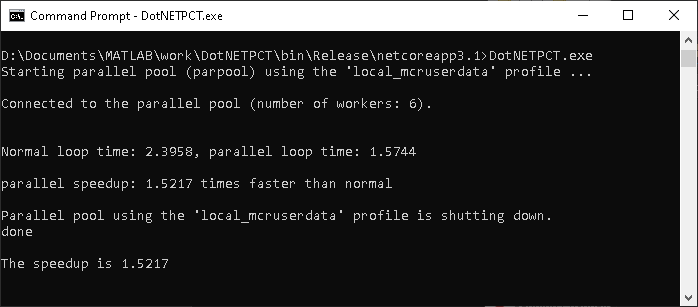## MATLAB Runtime User Data Interface

This feature allows data to be shared between a MATLAB® Runtime instance, the MATLAB code running on that MATLAB Runtime instance, and the wrapper code that created the MATLAB Runtime instance. Through calls to the MATLAB Runtime User Data interface API, you access MATLAB Runtime data by creating a per-instance associative array of `mxArray`s, consisting of a mapping from string keys to `mxArray` values. Reasons for doing this include, but are not limited to:

• You need to supply MATLAB Runtime profile information to a client running an application created with the Parallel Computing Toolbox™ software. Profiles may be supplied (and changed) on a per-execution basis. For example, two instances of the same application may run simultaneously with different profiles.

• You want to initialize MATLAB Runtime with constant values that can be accessed by all your MATLAB applications.

• You want to set up a global workspace — a global variable or variables that MATLAB and your client can access.

• You want to store the state of any variable or group of variables.

MATLAB Compiler SDK™ software supports a per-MATLAB Runtime instance state access through an object-oriented API. Access to a per-instance state is optional. You can access this state by adding `setmcruserdata.m` and `getmcruserdata.m` to your deployment project or by specifying them on the command line. Alternatively, you can use a helper function to call these methods as shown in Supplying Cluster Profiles for Parallel Computing Toolbox Applications.

### Supplying Cluster Profiles for Parallel Computing Toolbox Applications

Following is a complete example of how you can use the MATLAB Runtime User Data Interface as a mechanism to specify a cluster profile for Parallel Computing Toolbox applications.

#### Step 1: Write Your Parallel Computing Toolbox Code

1. Compile `sample_pct.m` in MATLAB.

This example code uses the cluster defined in the default profile.

The output assumes that the default profile is `local`.

```function speedup = sample_pct (n) warning off all; tic if(ischar(n)) n=str2double(n); end for ii = 1:n (cov(sin(magic(n)+rand(n,n)))); end time1 =toc; parpool; tic parfor ii = 1:n (cov(sin(magic(n)+rand(n,n)))); end time2 =toc; disp(['Normal loop times: ' num2str(time1) ... ',parallel loop time: ' num2str(time2) ]); disp(['parallel speedup: ' num2str(1/(time2/time1)) ... ' times faster than normal']); delete(gcp); disp('done'); speedup = (time1/time2); ```
2. Run the code as follows after changing the default profile to `local`, if needed.

`a = sample_pct(200)`

3. Verify that you get the following results:

```Starting parallel pool (parpool) using the 'local' profile ... connected to 4 workers. Normal loop times: 0.7587,parallel loop time: 2.9988 parallel speedup: 0.253 times faster than normal Parallel pool using the 'local' profile is shutting down. done a = 0.2530```

#### Step 2: Set the Parallel Computing Toolbox Profile

In order to compile MATLAB code to a .NET component and utilize Parallel Computing Toolbox, the `mcruserdata` must be set directly from MATLAB. There is no .NET API available to access the `MCRUserdata` as there is for C and C++ applications built with MATLAB Compiler SDK.

To set the `mcruserdata` from MATLAB, create an `init` function in your .NET class. This is a separate MATLAB function that uses `setmcruserdata` to set the Parallel Computing Toolbox profile once. You then call your other functions to utilize the Parallel Computing Toolbox functions.

Create the following `init` function:

```function init_sample_pct % Set the Parallel Profile: if(isdeployed) [profile] = uigetfile('*.settings'); % let the USER select file setmcruserdata('ParallelProfile', [profile]); end```

#### Step 3: Compile Your Function

You can compile your function from the command line by entering the following:

```mcc -W 'dotnet:netPctComp,NetPctClass' init_sample_pct.m sample_pct.m -T link:lib```

Alternately, you can use the Library Compiler app as follows:

1. Follow the steps in Generate .NET Assembly and Build .NET Application to compile your application. When the compilation finishes, a new folder (with the same name as the project) is created. This folder contains two subfolders: `distrib` and `src`.

 Library Name `netPctComp` Class Name `NetPctClass` Files to Compile `sample_pct.m` and `init_sample_pct.m`

Note

If you are using the GPU feature of Parallel Computing Toolbox, you need to manually add the PTX and CU files.

If you are using the Library Compiler app, click Add files/directories on the Build tab.

If you are using the `mcc` command, use the `-a` option.

2. To deploy the compiled application, copy the `for_redistribution_files_only` folder, which contains the following, to your end users.

Note

#### Step 4: Write the .NET Driver Application

After adding references to your component and to `MWArray` in your Microsoft® Visual Studio® project, write the following .NET driver application to use the component, as follows. See Integrate Simple MATLAB Function Into .NET Applicationfor more information.

Note

This example code was written using Microsoft Visual Studio 2008.

```using System; using MathWorks.MATLAB.NET.Utility; using MathWorks.MATLAB.NET.Arrays; using netPctComp; namespace PctNet { class Program { static void Main(string[] args) { try { NetPctClass A = new NetPctClass(); // Initialize the PCT set up A.init_sample_pct(); double var = 300; MWNumericArray out1; MWNumericArray in1 = new MWNumericArray(300); out1 = (MWNumericArray)A.sample_pct(in1); Console.WriteLine("The speedup is {0}", out1); Console.ReadLine(); // Wait for user to exit application } catch (Exception exception) { Console.WriteLine("Error: {0}", exception); } } } }```

The output is as follows:## SupportGet trial now